Question

# Rosie makes fudge using two ingredients, sugar and chocolate. She prefers to have more of both...

Rosie makes fudge using two ingredients, sugar and chocolate. She prefers to have more of both goods, but she also prefers similar quantities of each; otherwise the fudge is too sweet or too bitter. In fact, her preferences are given by the utility function U(S,C) = S 1/2 + C 1/2 .

A. What is Rosie’s preference between the bundle (4,16) and (16,4)? (Note that the consumption bundle is (# units of sugar, # units of chocolate)). What is Rosie’s preference between the bundle (4,16) and (9,9)? What is Rosie’s preference between the bundle (4,16) and (10,10)? Explain your choice in each case.

B. On a graph with sugar on the horizontal axis and chocolate on the vertical axis, draw the indifference curve that gives Rosie 6 units of utility (i.e., U = 6).

C. Find Rosie’s marginal utilities of sugar and chocolate. Does she have diminishing marginal utilities for them? How does that relate to the shape of the indifference curve in part (B)?

D. Write Rosie’s utility-maximizing tangency condition and solve for C. (You do not know prices so you will need to use PS and PC for the prices of sugar and chocolate, respectively.)

E. Write Rosie’s budget constraint. Use this and your work in part (D) to determine the demand for sugar. Is sugar a normal or inferior good for Rosie?

F. Assume that PS = PC = \$ 2. What bundle will Rosie consume with an income of \$ 20? What bundle will Rosie consume with an income of \$ 40? What bundle will Rosie consume with an income of \$ 60? Draw an income consumption curve (ICC) based on this data.

G. If war breaks out in the Middle East and sugar is rationed so that Rosie is limited to purchasing only 8 units of sugar, what bundles will she consume with an income of \$ 20? Did the restriction alter her consumption? Explain. What bundles will she consume with an income of \$ 40? Did the restriction alter her consumption? Explain. (2)

U= S(1/2)+C(1/2)

A. For the bundle (4,16) & (16,4)

(4,16) U= 4/2+16/2

= 2+8

=10

(16,4) U= 16/2+4/2

= 8+2

=10

In both cases the utility is same, Rosie will remain "Indifferent".

For bundle (4,16) & (9,9)

(4,16) U= 4/2+16/2

= 2+8

=10

(9,9) U= 9/2+9/2

= 4.5+4.5

= 9

As the utility is higher in first case but the amount of chocolate is more. And in second case the utility is 9( which is less than 10) but here the quantity of both ingrediants is same. Therefore, Rosie should choose 2nd bundle given her preference.

For bundle (4,16) & (10,10)

(4,16) U= 4/2+16/2

= 2+8

=10

(10,10) U= 10/2+10/2

= 5+5

= 10

In this case also she'll remain indifferent as the utility is same.But given that she wants equal proportion of both ingredients, she should go for the bundle (10,10)

B. See photo attached for graph

Given, U=S1/2+C1/2, following bundles should give utility = 6 for which Rosie will be indifferent

 Sugar (S) 4 8 0 Chocolate (C) 8 4 12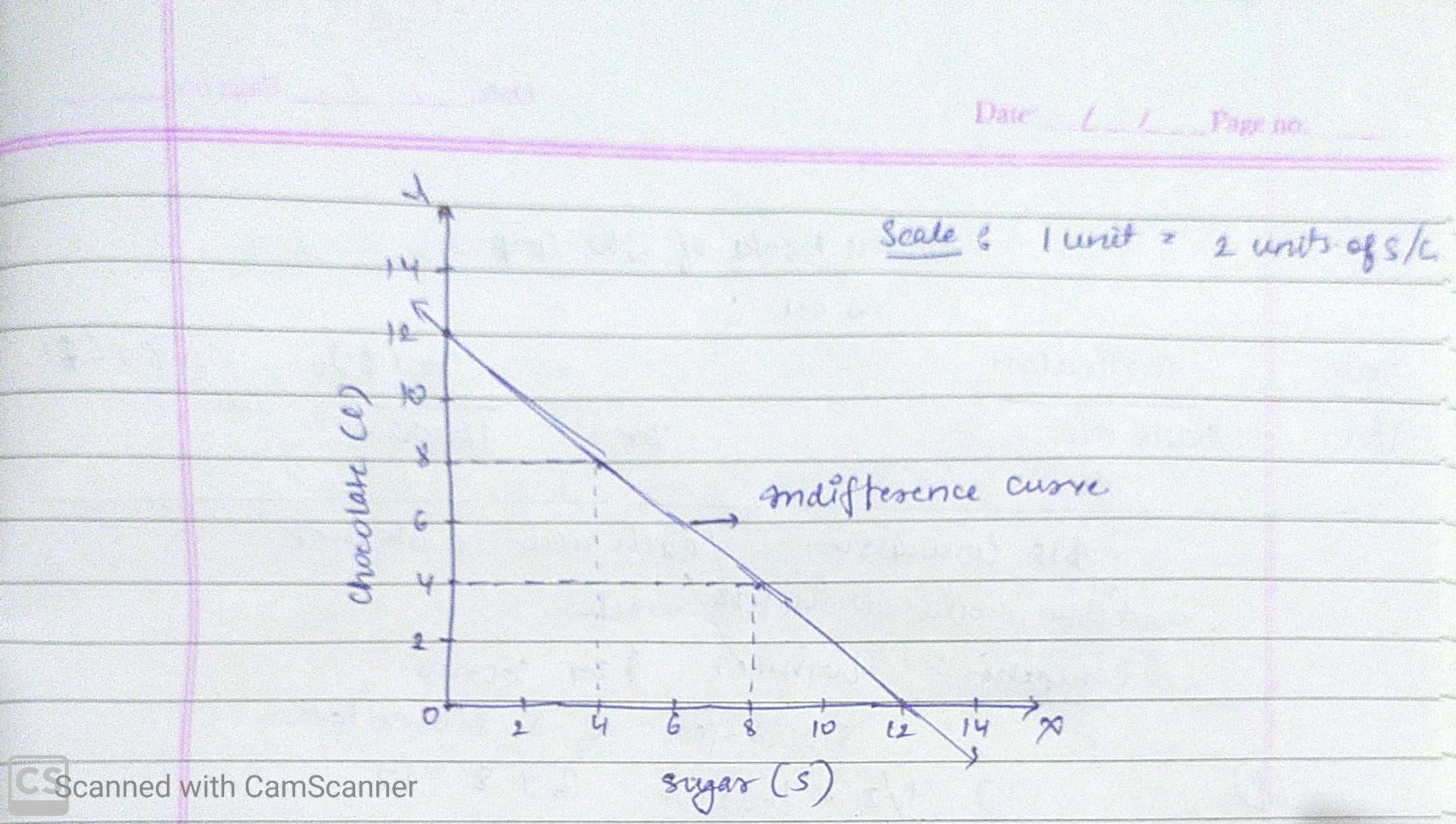#### Earn Coins

Coins can be redeemed for fabulous gifts.

Similar Homework Help Questions
• ### Exercise 3 For Sandra, coffee and sugar are perfect complements: she wants to consume exactly 2 g...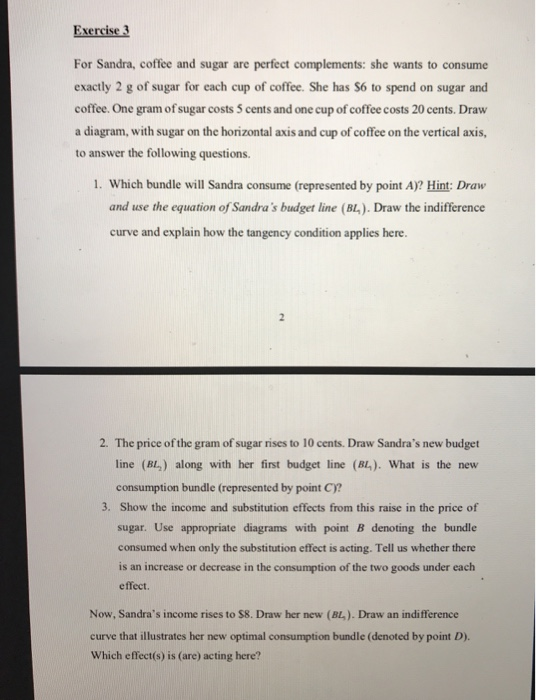Exercise 3 For Sandra, coffee and sugar are perfect complements: she wants to consume exactly 2 g of sugar for each cup of coffee. She has S6 to spend on sugar and coffee. One gram of sugar costs 5 cents and one cup of coffee costs 20 cents. Draw a diagram, with sugar on the horizontal axis and cup of coffee on the vertical axis, to answer the following questions. 1. Which bundle will Sandra consume (represented by point A)?...

• ### Exercise 3 For Sandra, coffee and sugar are perfect complements: she wants to consume exactly 2...Exercise 3 For Sandra, coffee and sugar are perfect complements: she wants to consume exactly 2 g of sugar for each cup of coffee. She has S6 to spend on sugar and coffee. One gram of sugar costs 5 cents and one cup of coffee costs 20 cents. Draw a diagram, with sugar on the horizontal axis and cup of coffee on the vertical axis, to answer the following questions. 1. Which bundle will Sandra consume (represented by point A)?...

• ### Lorelai's choice behavior can be represented by the utility function 11(xi, X2) = 0.91n(xi) + 0.1x2...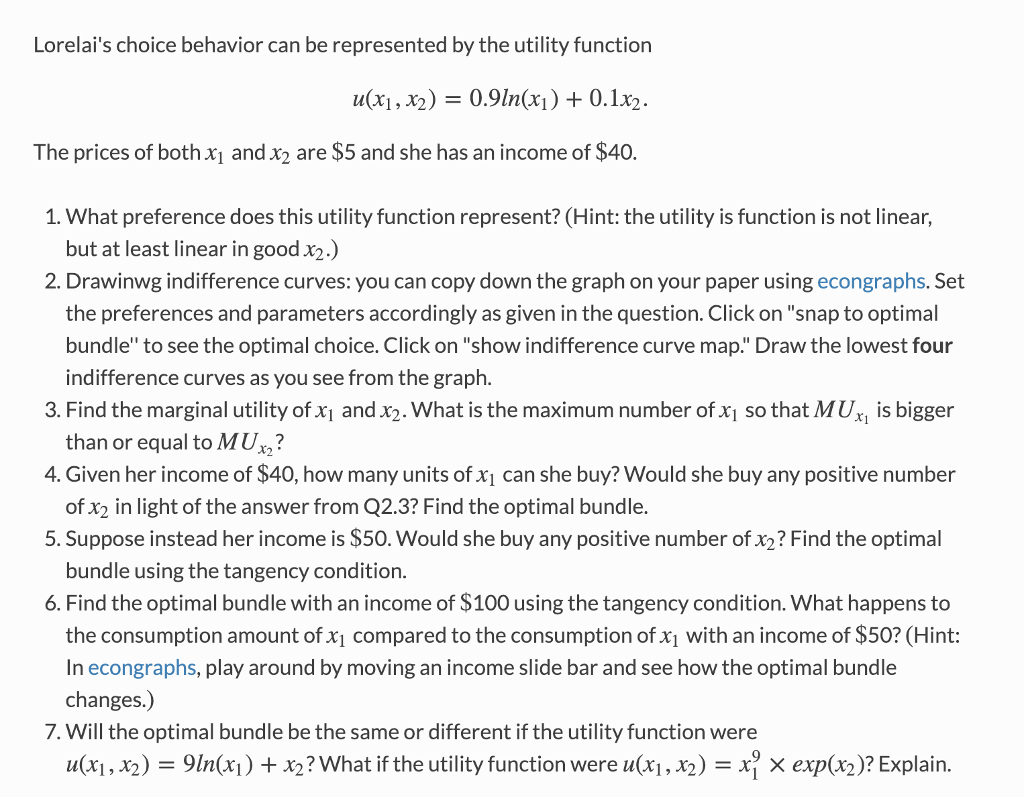Lorelai's choice behavior can be represented by the utility function 11(xi, X2) = 0.91n(xi) + 0.1x2 The prices of both x and x2 are \$5 and she has an income of \$40. 1. What preference does this utility function represent? (Hint: the utility is function is not linear, but at least linear in good x2.) 2. Drawinwg indifference curves: you can copy down the graph on your paper using econgraphs. Set the preferences and parameters accordingly as given in the...

• ### Lorelai's choice behavior can be represented by the utility function u(x1, 2) 0.9n(x)0.1x2. The prices of...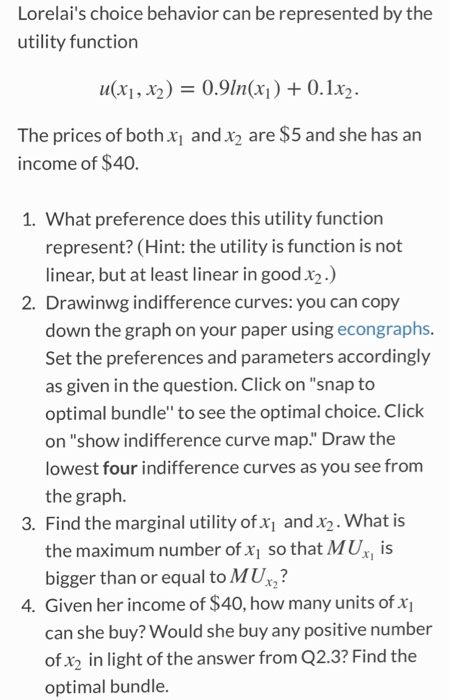Lorelai's choice behavior can be represented by the utility function u(x1, 2) 0.9n(x)0.1x2. The prices of both xi and x2 are \$5 and she has an income of \$40. 1. What preference does this utility function represent? (Hint: the utility is function is not linear, but at least linear in good x2) 2. Drawinwg indifference curves: you can copy down the graph on your paper using econgraphs. Set the preferences and parameters accordingly as given in the question. Click on...

• ### Question 2: Lorelai's choice behavior can be represented by the utility function u(x1, 2)0.9Inx)0.1x2 The prices...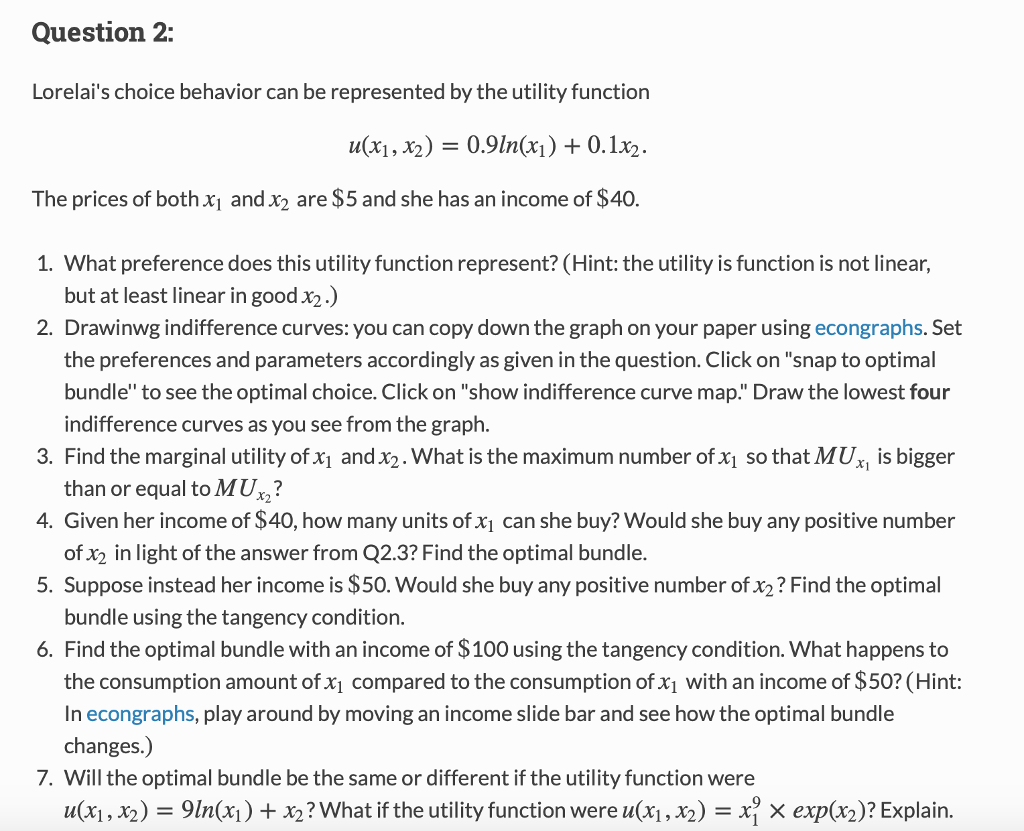Question 2: Lorelai's choice behavior can be represented by the utility function u(x1, 2)0.9Inx)0.1x2 The prices of both x1 and x2 are \$5 and she has an income of \$40. 1. What preference does this utility function represent? (Hint: the utility is function is not linear, 2. Drawinwg indifference curves: you can copy down the graph on your paper using econgraphs. Set but at least linear in good x2) the preferences and parameters accordingly as given in the question. Click...

• ### (Use this information to answer a, b, c below) Suppose Mary’s utility function for two goods...

(Use this information to answer a, b, c below) Suppose Mary’s utility function for two goods X and Y is given by: U(X,Y) = 3X1/2Y1/2 . Suppose consumption bundle A consists of 10 units of X and 30 units of Y, and consumption bundle B consists of 40 units of X and 20 units of Y. a. Consumption bundle A lies on a higher/lower/same indifference curve than consumption bundle B. Show computations. b. Compute Mary’s MRSxy at consumption bundle A....

• ### Question 1: Louis the retired Canadian lives on a fixed budget and consumes only two goods:...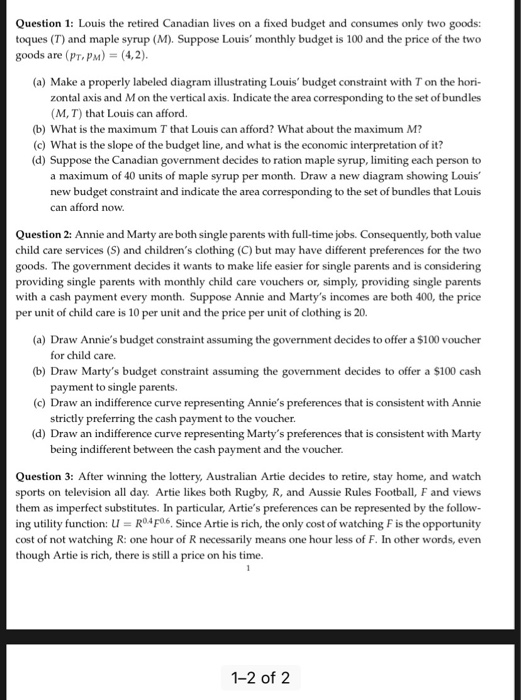Question 1: Louis the retired Canadian lives on a fixed budget and consumes only two goods: toques (T) and maple syrup (M). Suppose Louis monthly budget is 100 and the price of the two goods are (PT,PM) (4,2). (a) Make a properly labeled diagram illustrating Louis'budget constraint with T on the hori- zontal axis and M on the vertical axis. Indicate the area corresponding to the set of bundles (M, T) that Louis can afford. (b) What is the maximum...